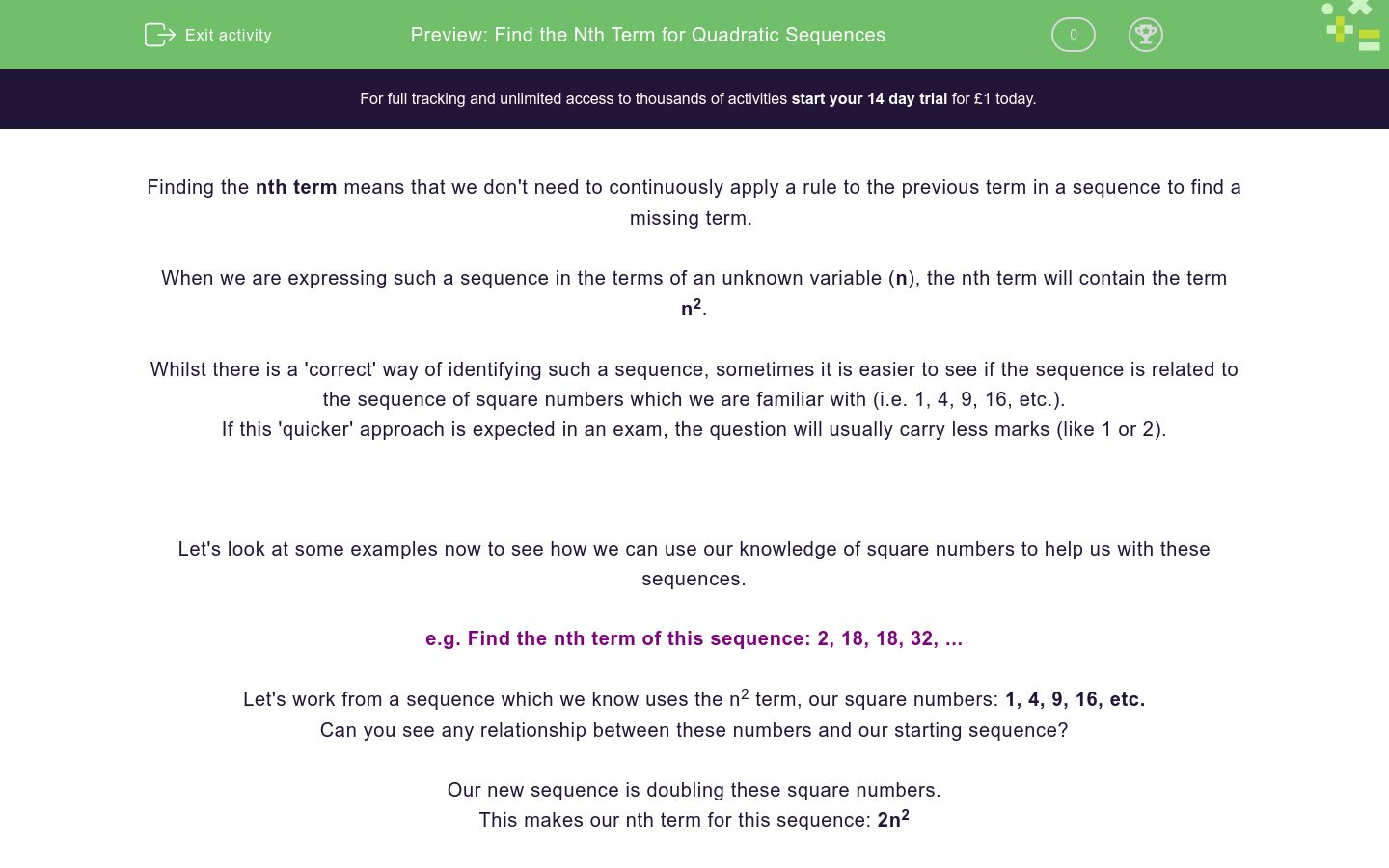# Nth Terms of Simple Quadratics

In this worksheet, students practise finding the nth term of a quadratic sequence.Key stage:  KS 4

GCSE Subjects:   Maths

GCSE Boards:   AQA, Eduqas, Pearson Edexcel, OCR

Curriculum topic:   Algebra

Curriculum subtopic:   Sequences

Difficulty level:### QUESTION 1 of 10

A quadratic sequence is a sequence where the nth term contains the term n2. While there is a 'correct' way of doing this, sometimes it is easier to see if the sequence is related to the sequence of square numbers (1, 4, 9, 16...),

The way we can check if this is the approach the examiner wants is to look how many marks it is worth, if it only carries 1/2 marks, use this apporach,

Example 1: Find the nth term of 2, 18, 18, 32...

Start by looking at the sequence 1, 4, 9, 16 (This has an nth term of n2)

You should notice that our sequence is double the square numbers. This makes our nth term 2n2

Example 2: Find the nth term of 3, 7, 11, 18...

Again, we can compare this with the square numbers and we notice that our sequence is just two more.

Our sequence would be n2 + 2

Example 3: Find the nth term of 19, 16, 11, 4

If we compare this to the square numbers, notice that they add up to 20.

This could be looked at as 'if I take the square numbers away from 20, I get my target'

This makes our nth term 20 - n2

What are the next two numbers in the sequence...

1, 4, 9, 16...

What is the 6th number in the sequence...

3, 12, 27, 48

What is the next number in the sequence...

-1, 2, 7, 14

25

27

23

Match the sequence with its nth term

## Column B

1, 4, 9, 16...
n2+5
3, 12, 27, 48...
3n2
6, 9, 14, 21
n2

The nth term for the sequence 3, 12, 27, 48 can be written as an2

Find the value of a

Which of these numbers will be in the sequence 0, 3, 8, 15...

24

80

50

37

63

120

The nth term of this sequence can be written as an2.

Find the value of a.

The nth term for the sequence 9, 6, 1, -6 can be written in the form a - bn2.

Find the value of a and b

What is the only number that appears in both the sequences defined by n2 and 2n-1

What is the nth term for...

4, 7, 12, 19...

n2 +3

3n2

3-n2

• Question 1

What are the next two numbers in the sequence...

1, 4, 9, 16...

EDDIE SAYS
A nice easy one to ease you in. This sequence is just the square numbers.
• Question 2

What is the 6th number in the sequence...

3, 12, 27, 48

108
EDDIE SAYS
If we compare these to the square numbers, we notice that they are 3 times bigger so our nth term is 3n2. The 6th number will therefore be 3 x 62
• Question 3

What is the next number in the sequence...

-1, 2, 7, 14

23
EDDIE SAYS
If you compare this to the square numbers, you can see they are 2 smaller. The answer we are looking for will be 2 less than the 5th square number.
• Question 4

Match the sequence with its nth term

## Column B

1, 4, 9, 16...
n2
3, 12, 27, 48...
3n2
6, 9, 14, 21
n2+5
EDDIE SAYS
Again, we need to compare these to the square numbers. n2+5 means 5 more than the square numbers 3n2 means 3 times the square numbers
• Question 5

The nth term for the sequence 3, 12, 27, 48 can be written as an2

Find the value of a

3
EDDIE SAYS
All of the numbers we are looking for are 3 times bigger than the square numbers
• Question 6

Which of these numbers will be in the sequence 0, 3, 8, 15...

24
80
63
120
EDDIE SAYS
Our sequence is 1 less than the square numbers, we can use this to work out that any number that is one less than a square number will be in the sequence.
• Question 7

The nth term of this sequence can be written as an2.

Find the value of a.

-
-1
- 1
EDDIE SAYS
Did you notice that these are just the square numbers with a minus sign in front of them? 1 must be -1 (although this could just be written as -)
• Question 8

The nth term for the sequence 9, 6, 1, -6 can be written in the form a - bn2.

Find the value of a and b

EDDIE SAYS
Comparing these to the square numbers shows they add up to 10. So to get our sequence, we have to take the square numbers away from 10.
• Question 9

What is the only number that appears in both the sequences defined by n2 and 2n-1

1
EDDIE SAYS
The easiest way to do this is to write out the sequences. n2: 1, 4, 9, 16 2n - 1: 1, 3, 5, 7
• Question 10

What is the nth term for...

4, 7, 12, 19...

n2 +3
EDDIE SAYS
Once again, just compare these to the square numbers. You should see they are all 3 more.
---- OR ----

Sign up for a £1 trial so you can track and measure your child's progress on this activity.

### What is EdPlace?

We're your National Curriculum aligned online education content provider helping each child succeed in English, maths and science from year 1 to GCSE. With an EdPlace account you’ll be able to track and measure progress, helping each child achieve their best. We build confidence and attainment by personalising each child’s learning at a level that suits them.

Get started